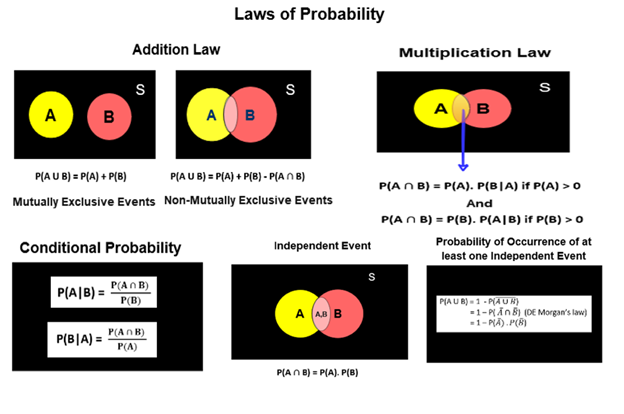## PublicationProbability

# Laws of Probability — A Primer for Data Scientists and Machine Learning Engineers

Last Updated on November 23, 2021 by Editorial Team

#### Author(s): Supriya Ghosh

Originally published on Towards AI the World’s Leading AI and Technology News and Media Company. If you are building an AI-related product or service, we invite you to consider becoming an AI sponsor. At Towards AI, we help scale AI and technology startups. Let us help you unleash your technology to the masses.

Real-life is a book that has probability in all its chapters.

What is probability?

Probability is a measure of uncertainty.

Real-world involves taking decisions at every step, but many times decisions have to be based on incomplete information wherein probability comes as a savior. This is one of the core reasons why probability is widely used in most Machine Learning applications.

Using probability, all elements of uncertainty can be modeled. Machine Learning Algorithms are designed using probability and they make decisions also using probability.

With this much introduction to probability, we can now jump straight to the topic.

This write-up will cover :

2. Multiplication Law of Probability

3. Conditional Probability

4. Independent Event and more

So let us start.

If A and B are non-mutually exclusive events (two events are considered to be non — mutually exclusive if they have chances of occurring at the same time)

And if A and B are mutually exclusive events (two events are considered to be mutually exclusive if they do not have chances of occurring at the same time)

The same law for three events can be represented as:

Non-mutually exclusive events

Mutually exclusive events

The above law can also be extended for more than three events if required.

Multiplication Law of Probability

For any two events A and B,

Also,

where P(B|A) is the conditional probability of B given that A has already

occurred and P(A|B) is the conditional probability of A given that B has

This same law can be extended for 3 or more events as below.

Conditional Probability

After going through the above Multiplication Law of Probability, it is very clear that the conditional probability can be represented as :

P(A|B) — the conditional probability of occurrence of A given that B has

already occurred i.e., here A occurs depending on the condition of B.

and

P(B|A) — the conditional probability of occurrence of B given that A has

already occurred i.e., here B occurs depending on the condition of A.

P(A|B) refers to sample space B and not S and P(B|A) refers to the sample space A and not S.

Note — P(A|B) is meaningful only when P(B) is not equal to 0 i.e. when the event B is not an impossible event and

P(B|A) is meaningful only when P(A) is not equal to 0 i.e. when the event A is not an impossible event.

Let’s understand conditional probability through an example.

Consider a card is drawn from a pack of 52 playing cards. Let A be the event of drawing a black color queen card. Then of course

P(A) = 2/52 as we have 2 black queen cards in a pack of 52 cards.

Now let B be the event of drawing a card of a spade.

P(B) = 13/52

since there are 13 spades in a total of 52 cards and we know that B has already occurred hence there are 13 exhaustive cases and not 52 as earlier. From amongst these 13 cards of spade, there is 1 black color queen card and hence the probability of having black color queen card given that it is a card of spade i.e., P(A|B) = 1/13, which is the conditional probability of A given that B has already occurred.

Independent Event

Events are said to be independent if the occurrence or non-occurrence of any one event is not affected by the occurrence or non-occurrence of other events.

A very common example, in this case, is that of a coin, i.e., if a coin is tossed a certain number of times, then the occurrence of the head in any trial is not affected by any other trial i.e., all the trials are said to be independent.

One more important point to look at here is — Two events A and B are said to be independent if and only if P(B|A) = P(B) i.e., even if A has already occurred, it does not affect the probability of B. One example to consider is :

let A be the event of getting head in the 4th toss of a coin and B be the event of getting head in the 5th toss of the coin. Then the probability of getting head in the 5th toss is 1/2, irrespective of whether the outcome of the 4th toss is known or not i.e., P(B|A) = P(B).

Now let’s represent Multiplication Law for Independent Events:

This is because if A and B are independent then P(B|A) = P(A) and P(A|B) = P(B).

Similarly, if A, B, and C are three independent events, then

This law can be extended for more than three events also.

One very important point to note here is that mutually exclusive events cannot be independent because, if two events are mutually exclusive then they do not occur simultaneously and hence they are not independent.

One more important law in probability is

If A and B are independent events, then the probability of occurrence of at least one of the events is given by:

Now let us summarize the main points covered.

Summary

Hope this helped.

You can follow me on medium as well asLaws of Probability — A Primer for Data Scientists and Machine Learning Engineers was originally published in Towards AI on Medium, where people are continuing the conversation by highlighting and responding to this story.

Join thousands of data leaders on the AI newsletter. It’s free, we don’t spam, and we never share your email address. Keep up to date with the latest work in AI. From research to projects and ideas. If you are building an AI startup, an AI-related product, or a service, we invite you to consider becoming a sponsor.

Published via Towards AI